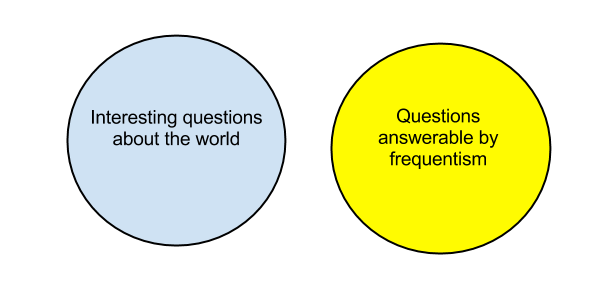Feeds:
Posts

## Interesting questions about the world

A great post by Ted Bunn on the difference between Bayesian and frequentist approaches to probability, summarised in this marvellous plot:Highlight: “Frequentism simply refuses to answer questions about the probability of hypotheses. … In frequentist analyses, all probabilities are of the form P(data | hypothesis), pronounced “the probability that the data would occur, given the hypothesis.” Frequentism flatly refuses to consider probabilities with the hypothesis to the left of the bar — that is, it refuses to consider the probability that any scientific hypothesis is correct.”

### 2 Responses

1. on June 18, 2013 at 2:07 am | ReplyunkleE

I trained as a mere engineer and hydrologist, so I studied statistics for only one year (though I did get a distinction – not sure how!), so what would I know? But the Bunn article quite flabbergasts me. Is statistics so unclear a discipline?

I understand Bunn to be saying that Bayesian stats goes from data and an old hypothesis to a new hypothesis, whereas frequentist stats goes from a hypothesis (or a known) to predictions about data. If that is broadly so, how can professors of statistics be so confused about it???

Or am I, in my naivety, simply missing the subtleties?

2. on June 19, 2013 at 11:52 pm | ReplyBrendon J. Brewer

I loved that post too. Getting ready to teach our big undergrad Bayesian course again, it got me all fired up.